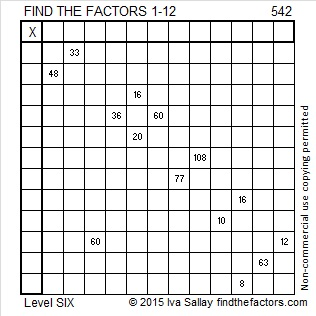# 542 and Level 6

Here are some ways to make 542 by adding together exactly three square numbers.

• 542 = (21^2) + (10^2) + (1^2)
• 542 = (19^2) + (10^2) + (9^2)
• 542 = (18^2) + (13^2) + (7^2)
• 542 = (15^2) + (14^2) + (11^2)Print the puzzles or type the solution on this excel file: 12 Factors 2015-06-29

—————————————————————————————————

• 542 is a composite number.
• Prime factorization: 542 = 2 x 271
• The exponents in the prime factorization are 1 and 1. Adding one to each and multiplying we get (1 + 1)(1 + 1) = 2 x 2 = 4. Therefore 542 has exactly 4 factors.
• Factors of 542: 1, 2, 271, 542
• Factor pairs: 542 = 1 x 542 or 2 x 271
• 542 has no square factors that allow its square root to be simplified. √542 ≈ 23.280893—————————————————————————————————This site uses Akismet to reduce spam. Learn how your comment data is processed.